Journal of Measurements in Engineering

Published: 31 December 2018

# Experimental investigations on permeability and damage mechanism of cemented soil in acidic and alkali environments

Chen Sili1
Hou Rui2
Zhang Jingyu3
Wang Junxiang4
1, 2, 3, 4School of Architecture and Civil Engineering, Shenyang University of Technology, Shenyang, 110870, China
Corresponding Author:
Chen Sili
Views 304

#### Abstract

Cemented soil impermeable projects directly or indirectly contact an underground corrosive environment in most cases, which could cause reduced impermeability and malfunction of the cemented soil. Laboratory simulation permeability tests of cemented soil were conducted under different cement ratios in acidic and alkali environments. The variation in the permeability coefficient of the cemented soil was studied under different curing times, pH, and cement contents. Using scanning electron microscopy (SEM) and energy spectrum analysis, the permeability of cemented soil in acidic and alkali environments was studied at the microstructure level. The test results show that an acidic environment has a major effect on the permeability coefficient of cemented soil with a low cement content, and a greater cement content offers increased resistance to acid erosion. In an alkali environment of pH 9-13, the permeability coefficient of the cemented soil gradually decreases as the curing time increases. The research results provide technical parameters for the impermeability and durability design and application of cemented soil in impermeable projects, which has important engineering implications.

## 1. Introduction

Cemented soil is a stabilized soil formed by mixing natural soft soil with cement paste (or powder) in a certain proportion that mainly depends on the mechanical stirring [1, 2]. With the advantages of simplicity in construction, a short construction period, low permeability, and high strength [3, 4], cemented soil is widely used in slope retaining walls, slope reinforcement, dam foundation consolidation, subgrade engineering, reservoirs and shallow lake liners, foundation pit seepage-proof engineering in waterproof curtains, anti-seepage walls, and others [5-8]. However, most cemented soil used in impermeable engineering applications makes direct contact with a corrosive medium, e.g., groundwater often contains a certain concentration of Mg2+, Ca2+, SO42-, Cl-, and CO32-. These corrosive solutions can lead to degradation in the strength and durability of cemented soil materials [9, 10].

In recent years, researchers have examined the properties of cemented soil from many aspects. Lohani et al.  studied the influence of water content and curing age on the compressive strength of cemented soil. Bushra et al.  studied the influence of different cement contents and confining pressures on the shear strength of cemented soil. Arvind et al.  analyzed the microstructure of cemented soil with X-ray diffraction (XRD) and scanning electron microscopy (SEM) to study the strength properties of fly ash cement composite soil. Festugato L. et al.  studied the compressive and tensile strength of fiber-reinforced cemented soil and established the functional relationship between compressive strength, flexural strength, and fiber length. Most researchers adopted the standard environmental curing cemented soil permeability tests and studied the effect of curing age, cement content, and additives on permeability. Chen et al. [15-18] studied the mechanical properties and permeability of cemented soil under freezing and thawing conditions. Fan et al.  studied the impact of water qualities on the permeability of cemented soil. Yuan et al.  studied the permeability coefficient of cemented soil with the change in confining pressure. Dong et al.  used an orthogonal experiment toanalyze the variation of the permeability coefficient with different cement, bentonite, and fly ash content. Ji J. L. et al.  studied the permeability characteristics and prediction model of cemented soil containing sand. It is well known that most cemented soil in impermeable projects makes contact with groundwater . Most groundwater contains corrosive media, which can lead to a reduction in the impermeability of cemented soil, and even failure. In a soil layer containing corrosive groundwater, attention should be paid not only to the safe use of cemented soil but to the effect of construction procedures . Especially in construction using powdered jet pile that uses groundwater directly, groundwater pollution has a major impact on the pile quality [25-27]. Researchers are paying increased attention to the mechanical properties of cemented soil and carrying out experiments in specific chemically-polluted environments, with different chemical solutions, concentrations, pH values, etc. [28-30].

However, there are few reports on permeability of cemented soil in a corrosive environment. In practical engineering, contact with a corrosive medium by cemented soil is inevitable. To address this engineering reality, a permeability test of cemented soil under different acidic and alkali environments was carried out, and the variation of the permeability coefficient of the cemented soil was investigated. Furthermore, the permeability mechanism of the cemented soil in acidic and alkali environments was analyzed at the microscopic level. This work provides technical parameters of impermeability and durability for the design and application of cemented soil impermeable projects, which has major engineering significance.

## 2. Testing program

To analyze the effect of acidic and alkali environments on the permeability of cemented soil, laboratory permeability tests of cemented soil were carried out with different cement contents and pH values, with curing times of 7 d, 15 d, 30 d, and 60 d. The undisturbed soil used in the experiment was silt taken from a road base in Shenyang City, LiaoNing. The soil’s natural state was soft-plastic. The main physical and mechanical indices of the silts are provided here: 16.5 % natural moisture content, 18.8 kN/m3 natural gravity, 20.0 % liquid limit, 16.3 % plastic limit, 3.75 plasticity index, and 0.07 liquid index. The experimental soil was air dried, crushed, and passed through a 5 mm sieve.

### 2.1. Mixing proportion of cemented soil

Portland slag cement 32.5 was used in the test. Cement and silt were placed in a blender and mixed evenly for content of 7 %, 10 %, and 13 %. The mixture was poured into molds with an upper diameter of 80 mm, bottom diameter of 70 mm, and height of 40 mm. The cemented soil specimens were well compacted by vibration, numbered, demolded after 24 hours, and placed in preconfigured solutions of different pH values for curing. The laboratory temperature was between 22 °C and 28 °C. The specimens under curing are shown in Fig. 1(a).

### 2.2. Permeability tests of cemented soil in different pH environments

(1) Hydrochloric acid and sodium hydroxide were used to configure different pH solutions.

(2) After demolding, cemented soil specimens were cured in different pH solutions (pH = 2, pH = 4, pH = 9, pH = 10, pH = 13). The pH value is monitored daily during curing. When it fluctuated more than 0.5, hydrochloric acid or sodium hydroxide is immediately added to the solution to maintain a constant pH value.

(3) According to Cemented Soil Mixing Proportion Design Procedures (JGJT 233-2011) the experimental requirements, permeability tests of cemented soil specimens were carried out. The main equipment was a penetration apparatus as shown in Fig. 1(b).

Fig. 1Curing of cemented soil specimens and penetration apparatus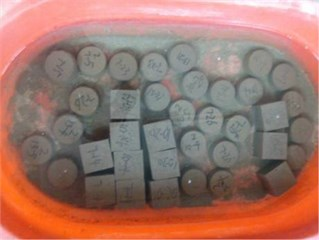a) Curing of specimens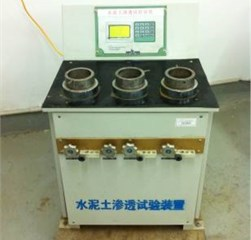b) Penetration apparatus

## 3. Test results and analysis

### 3.1. Calculation of permeability coefficient of cemented soil

The equation for the permeability coefficient of cemented soil is:

1
${k}_{T}=\frac{100{\gamma }_{w}Vh}{pAt},$

where ${k}_{T}$ is the permeability coefficient of the cemented soil (cm/s) at water temperature $T$ °C, $V$ is the exudation water volume (ml) for time interval $t$, $h$ is the height of the specimen (cm), $p$ is the applied seepage pressure (MPa), $A$ is the cross-sectional area of the specimen (cm2), $t$ is the time interval (s), and ${\gamma }_{w}$ is the water gravity (N/cm3).

The permeability test was conducted under the standard 20 °C water temperature. The permeability coefficient at the standard temperature is:

2
${k}_{20}={k}_{T}×\frac{{\eta }_{T}}{{\eta }_{20}},$

where ${k}_{20}$ is the permeability coefficient of the specimen (cm/s) at the standard temperature, is the dynamic viscosity coefficient at a water temperature of $T$ °C (kPa·s), and ${\eta }_{T}$ is the dynamic viscosity coefficient at 20 °C (kPa·s),These specific values were given by JGJT 233-2011 cemented soil mixing proportion design procedures.

### 3.2. Effect of different curing times on permeability of cemented soil

Permeability tests of cemented soil specimens were respectively conducted after curing periods of 7 d, 15 d, 30 d, and 60 d. In an acidic environment (pH = 2), the permeability coefficient of cemented soil with 7 % cement content decreased gradually over the initial curing time (7-30 d). Its permeability coefficient reached its minimum in 30 d, decreasing by 67.42 % from its value after 7 d curing. After that, the permeability coefficient increased gradually, showing a U-shaped change, increasing by 14.25 % in 60 d relative to 7 d. The results indicate that in an acidic environment, cemented soil with low cement content cannot resist acidic corrosion. In contrast, the permeability coefficient of cemented soil with 10 % or 13 % cement content decreased gradually with curing time, relative to 7 d by 32.05 % and 85.31 %, respectively, at 60 d.

In acidic environment (pH = 4), the permeability of cemented soil presented the same trend as for pH = 2. As shown in Fig. 3, the permeability coefficient of cemented soil with 7 % cement content reached a minimum in 30 d, decreasing by 72.88 % relative to 7 d. The permeability coefficient increased gradually after that, showing a V-shaped change. In contrast, the permeability coefficients of cemented soil with 10 % and 13 % cement content decreased gradually, by 71.48 % and 94.65 %, respectively, at 60 d.

Fig. 2Variation of permeability coefficient in the environment of pH = 2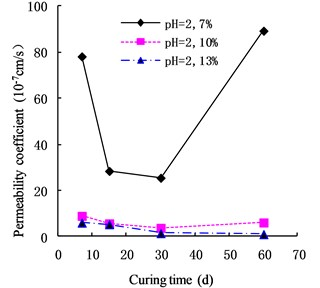Fig. 3Variation of permeability coefficient in the environment of pH = 4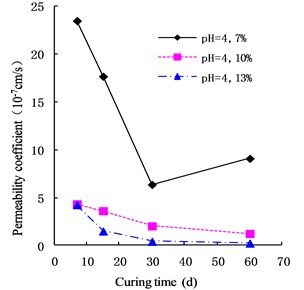The variation of the permeability coefficient of cemented soil with different cement content in different alkaline environments (pH = 9, pH = 10, and pH = 13) is shown in Figs. 4, 5, and 6. In the alkaline environment of pH = 9, the permeability coefficient of cemented soil with 7 %, 10 %, and 13 % cement content decreased by 87.67 %, 89.81 %, and 97.73 % respectively, in 60 d. In the alkaline environment of pH = 10, the permeability coefficient decreased by 96.98 %, 94.30 %, and 95.35 %, respectively, over the same time. In the alkaline environment of pH = 13, it decreased by 96.80 %, 93.58 %, and 98.50 %, respectively in that time. These results show that the impermeability of cemented soil improves with increasing curing time in an alkaline environment.

Fig. 4Variation of permeability coefficient in the environment of pH = 9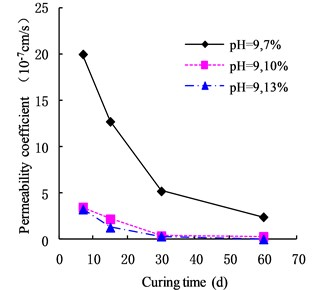Fig. 5Variation of permeability coefficient in the environment of pH = 10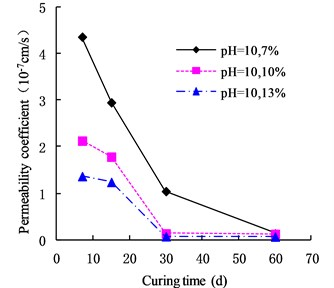### 3.3. Effect of different pH values on permeability of cemented soil

As shown in Figs. 7, 8, and 9, the permeability coefficients of cemented soil with 7 %, 10 %, and 13 % cement content decreased gradually as the pH value increased. After curing periods of 7 d, 15 d, 30 d, and 60 d, from an acidic environment (pH = 2) to an alkaline environment (pH = 13), the permeability coefficient decreased gradually. In an alkaline environment (pH = 13), the permeability coefficients showed that ${K}_{7d}>{K}_{15d}>{K}_{30d}>{K}_{60d}$, which indicates that the alkaline conditions are conducive to the hydration reaction and promote the inner compaction against pores, resulting in improvement of cemented soil’s impermeability. Therefore, the acidic environment has a corrosive effect on the cemented soil. The lower the pH, the larger the permeability coefficient of cemented soil is.

Fig. 6Variation of permeability coefficient in the environment of pH = 13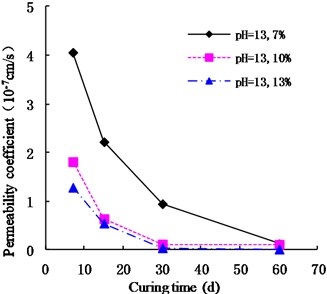Fig. 7Variation of permeability coefficient with 7 % cement content with pH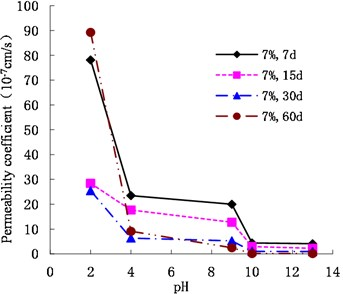Fig. 8Variation of permeability coefficient with 10 % cement content with pH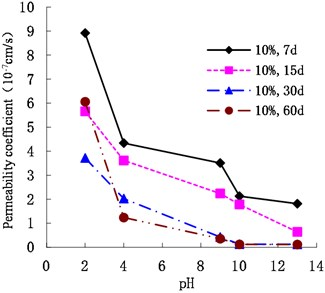Fig. 9Variation of permeability coefficient with 13 % cement content with pH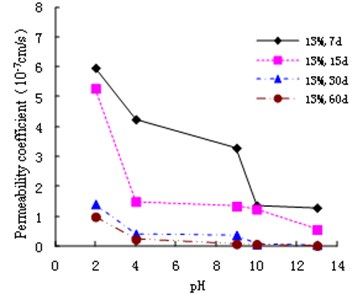### 3.4. Effect of different cement content on permeability of cemented soil

As shown in Figs. 10 and 11, in an acidic environment (pH = 2 and pH = 4), the permeability coefficient of cemented soil with different curing ages decreased gradually with increasing cement content. In acidic environment (pH = 2), at the curing age of 7 d, 15 d, 30 d, and 60 d, the permeability coefficient of cemented soil with 13 % cement content decreased by 92.36 %, 81.41 %, 94.45 %, and 98.90 % respectively, compared to cemented soil with 7% cement content. Similarly, in acidic environment (pH = 4), it decreased by 81.92 %, 91.57 %, 93.49 %, and 97.50 %, which shows that in an acidic environment, increasing the cement content increases cemented soil’s resistance to acidic corrosion.

As shown in Figs. 12, 13, and 14, in an alkaline environment (pH = 9, pH = 10, and pH = 13), the permeability coefficient of cemented soil with different curing ages decreased gradually with increasing cement content. In an alkaline environment (pH = 9), at curing ages of 7 d, 15 d, 30 d, and 60 d, the permeability coefficient of cemented soil with 13 % cement content decreased by 83.54 %, 89.50 %, 92.84 %, and 96.97 %, respectively, compared to cemented soil with 7 % cement content. Similarly, in alkaline environments with pH = 10 and pH = 13, the permeability coefficient decreased respectively by 68.74 %, 58.04 %, 93.33 %, and 51.91 % for pH = 10 and 68.27 %, 75.15 %, 94.98 %, and 85.18 % for pH = 13. This shows that in an alkaline environment, an increase in cement content is advantageous to hydration reaction and impermeability of cemented soil.

Fig. 10Variation of permeability coefficient with cement content in the environment of pH = 2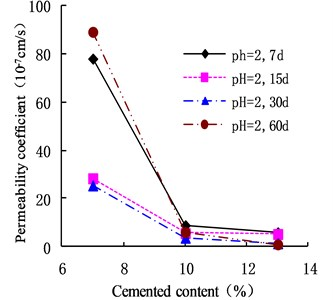Fig. 11Variation of permeability coefficient with cement content in the environment of pH = 4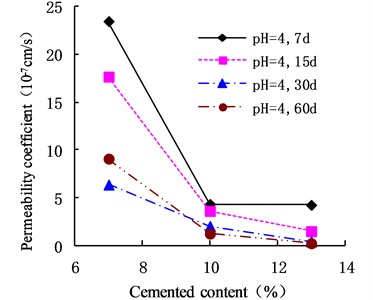Fig. 12Variation of permeability coefficient with cement content in the environment of pH = 9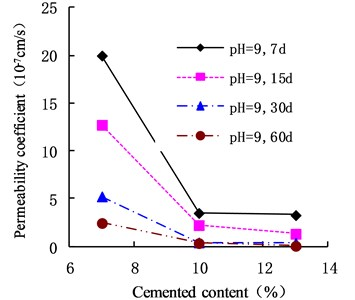Fig. 13Variation of permeability coefficient with cement content in the environment of pH = 10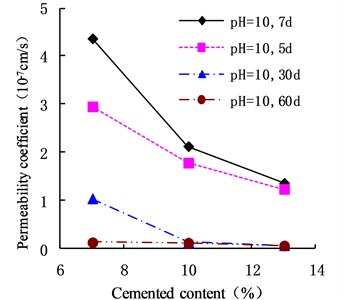Fig. 14Variation of permeability coefficient with cement content in the environment of pH = 13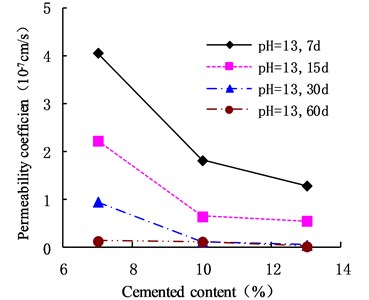## 4. Analysis of effect of acidic and alkaline environments on permeability of cemented soil

### 4.1. Macro damage analysis of pH effect on cemented soil

The surface damage of cemented soil specimens is shown in Fig. 15 for curing periods of 60 d in pH = 2, pH = 4, pH = 9, pH = 10, and pH = 13. Among these, in the acidic environment, the cemented soil specimens with 7 % cement content were seriously damaged compared to others, and their surfaces appeared loose. The surface of the cemented soil specimens with 13 % cement content changed very little. These observations indicate that acidic environment has an effect on cemented soil specimens with lower cement content. In the alkaline environment, the surface of the specimens did not appear broken or loose, and the cemented soil specimens with different cement content changed very little. These observations indicate that the alkaline environment does not cause damage to the specimens.

Fig. 15The surface damage of specimens with different cement content in different environment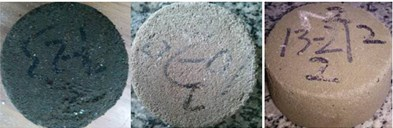pH = 2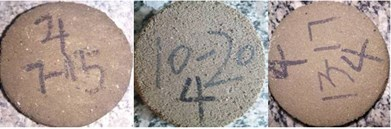b) pH = 4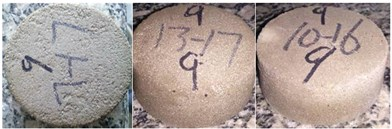c) pH = 9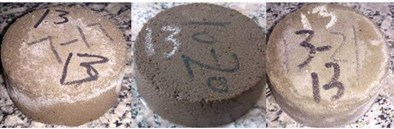d) pH = 13

### 4.2. Analysis of micro-mechanism of pH effect on cemented soil

Using scanning electronic microscopy (SEM) and energy spectrum analysis, specimens magnified 3,000 times are presented in Figs. 16, 17, and 18. The pictures show that cement gel existed between the particle surface and intergranular when cured in acidic environment. Sliced surfaces of specimens with 7 % and 10 % cement content showed honeycomb hydrate crystallization, which was attached to a fibrous gel material surface generated by the hydration reaction of cemented particles. When cured in acidic environment, specimen sections with 7 % cement content showed a larger loose region. Specimen sections with 10 % cement content appeared relatively more compact in the loose zone with reduced pore size. Specimen sections with 13 % cement content was full of small particles, in which small particles and the overall slice are bonded with each other. The sliced surface had a small number of needle crystals. The cemented soil had a granular-mosaic-gel structure. The skeleton was in the shape of a particle. There were many tiny pores in the connection position between the skeleton particles and gel, most with a long, flat shape and uneven size, and they were connected to each other. The pore sizes in cemented soil sections with a cement content of 13% decreased relative to those of 7 % and 10 % cement content. This indicates that an increase in cement content can improve the resistance to acidic corrosion. When cemented soil was cured in acidic environment (pH = 2 and pH = 4), the cemented hydration products were decomposed by H+, which caused the decomposition of the internal fillers. Specimens of Fe2O3, Al2O3, K2O, and Na2O oxide were also decomposed by H+, leading to internal looseness and the appearance of a large number of pores inside the specimens. These macroscopic effects reflect that the surface of the specimens becomes loose (as shown in Fig. 15), the compressive strength diminishes, and the permeability increases.

As shown in Figs. 19, 20, and 21, in the alkaline environment of pH = 10, sections of soil with 7 % cement content magnified 3,000 times show that these cemented soil sections have no obviously loose regions compared to those in a strongly acidic curing environment. Particles were clearly distinct, and many needle crystals were attached to the particles. These particles showed solid cementation by needle crystals constituting a skeleton embedded in a contact structure. The strength of specimens improved. This shows that in contrast to an acidic environment, the internal cement hydration reaction of specimens was sufficient in the alkaline environment. Under the same curing condition, sections with 10 % and 13 % cement content were relatively denser, hydrate crystallization increased significantly, and the crystalline structure was bulkier. Small particles and needle crystals consisted of large particles in a rod-like system structure distributed in the soil. Sections with 13 % cement content were relatively denser. Therefore, hydration was increasing, which intensified cementation. Consequently, a granular-mosaic-gel structure is formed.

Fig. 16The scanning electronic microscopy and energy spectrum of 7 % cement content in the environment of pH = 2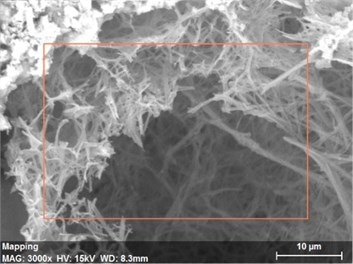a) The scanning electronic microscopy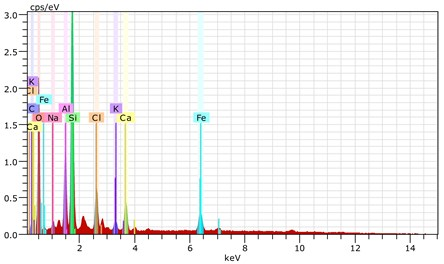b) The energy spectrum

Fig. 17The s canning electronic microscopy and energy spectrum of 10 % cement content in the environment of pH = 2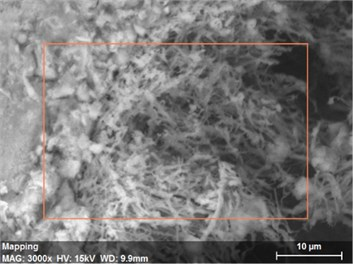a) The scanning electronic microscopy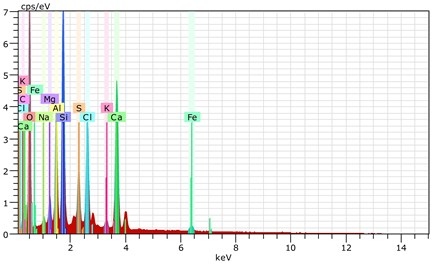b) The energy spectrum

Fig. 18The scanning electronic microscopy and energy spectrum of 13 % cement content in the environment of pH = 2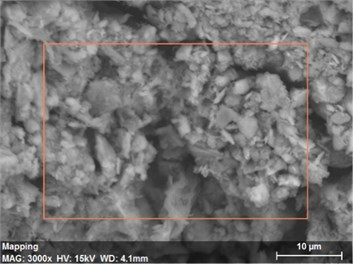a) The scanning electronic microscopy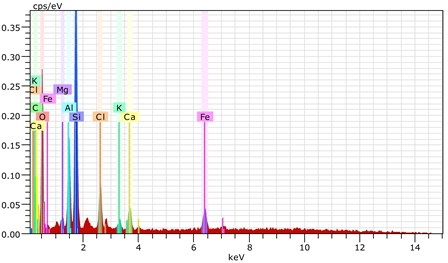b) The energy spectrum

Fig. 19The scanning electronic microscopy and energy spectrum of 7 % cement content in the environment of pH = 10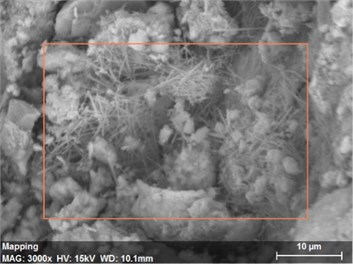a) The scanning electronic microscopy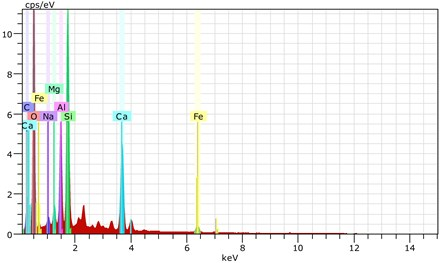b) The energy spectrum

Fig. 20The scanning electronic microscopy and energy spectrum of 10 % cement content in the environment of pH = 10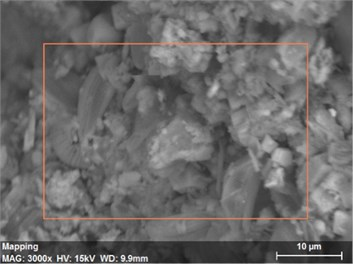a) The scanning electronic microscopy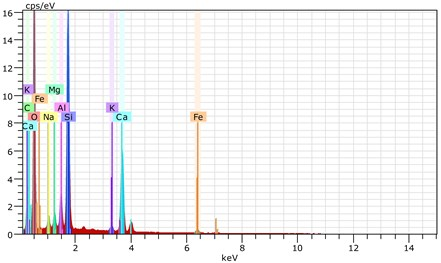b) The energy spectrum

As shown in Figs. 22, 23, and 24, in the alkaline environment of pH = 13, sections with 7 % cement content magnified 3,000 times showed that the degree of density follows 13 % > 10 % > 7 %. Compared to other environmental conditions, the degree of density of the cement content sections increased with different size of particles in mutual cementation. In sections with 7 % cement content, the large and small particles glued to each other and the porosity decreased significantly. The sections with 10 % and 13 % cement content did not show obvious porosity, indicating that the increase in pH was advantageous to cement hydration. The corresponding macroscopic manifestation was the enhancement of both the unconfined compressive strength and impermeability of specimens.

Fig. 21The scanning electronic microscopy and energy spectrum of 13 % cement content in the environment of pH = 10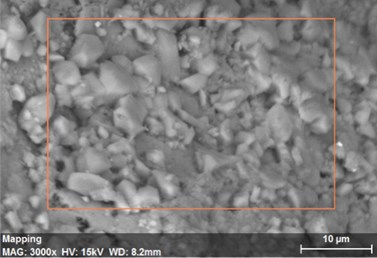a) The scanning electronic microscopy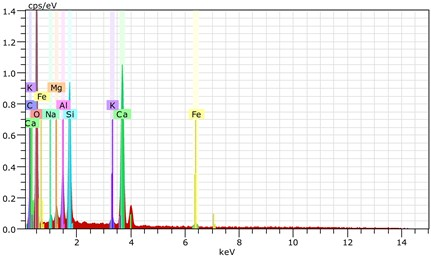b) The energy spectrum

Fig. 22The scanning electronic microscopy and energy spectrum of 7 % cement content in the environment of pH = 13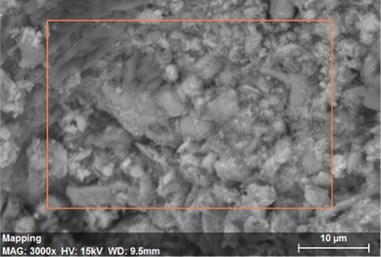a) The scanning electronic microscopy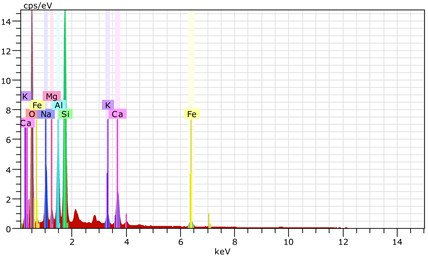b) The energy spectrum

Fig. 23The scanning electronic microscopy and energy spectrum of 10 % cement content in the environment of pH = 13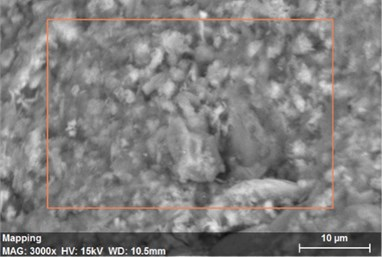a) The scanning electronic microscopy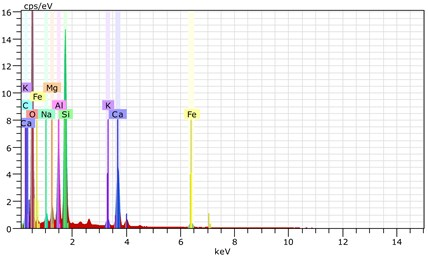b) The energy spectrum

Fig. 24The scanning electronic microscopy and energy spectrum of 13 % cement content in the environment of pH = 13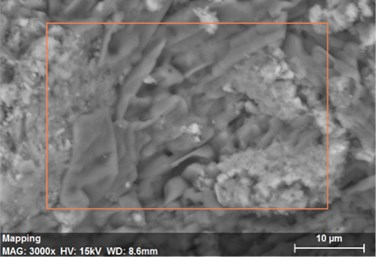a) The scanning electronic microscopy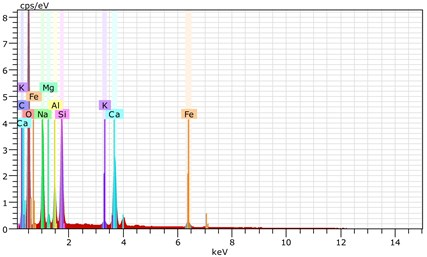b) The energy spectrum

## 5. Conclusions

This paper presents a series of laboratory simulation tests that measured the permeability of soils with different cement ratios. SEM and energy spectrum analyses were also carried out for the cemented soil under acidic and alkali environments. The main conclusions are as:

1) In acidic environments of pH = 2 and 4, the permeability coefficient of cemented soil with a 7 % cement content gradually decreased in the initial curing period between 7 and 30 days, reaching a minimum at 30 days. The permeability coefficient then increased gradually. However, the permeability coefficients of cemented soil with 10 % and 13 % cement contents gradually decreased with increased curing time. An acidic environment has a huge impact on the permeability coefficient of cemented soil with low cement content. The increase in cement content is beneficial to resist the corrosion of cemented soil due to acidity.

2) In the alkaline environments of pH = 9, 10, and 13, the permeability coefficient of cemented soil gradually decreased with increased curing time, and its impermeability was gradually enhanced.

3) Whether in an acidic or alkaline environment, the permeability coefficient of cemented soil gradually decreased with increased cement content, and its impermeability was gradually enhanced.

4) With 7 %, 10 %, and 13 % cement contents, the permeability coefficient of cemented soil gradually decreased as the pH increased (pH = 2 → 4 → 9→ 10 → 13), and its permeability coefficient gradually decreased.

5) The analysis of the damage mechanism showed that in an acidic environment, cemented soil with low cement content showed surface loosening, the cemented hydration was insufficient, and the hydrate crystallization formed a honeycomb structure. The internal pores were interconnected. The macroscopic manifestation corresponding to the above is that of an increase in the permeability. With increases in cement content and pH, hydrate crystallization increased significantly. Small particles and needles consisting of large particles were observed to be distributed in the soil. The structure unit body showed a reunion structure, playing a positive role in cementation. A granular-mosaic-gel structure emerged with a macroscopic manifestation of improved impermeability.

#### Acknowledgements

This work was financially supported by the National Natural Science Foundation of China (Issue 51608332, 51279109).